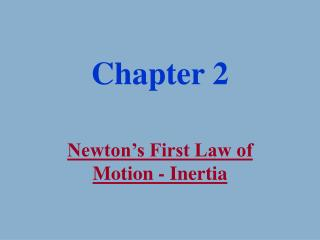Download PresentationChapter 2

# Chapter 2 - PowerPoint PPT PresentationDownload Presentation## Chapter 2

- - - - - - - - - - - - - - - - - - - - - - - - - - - E N D - - - - - - - - - - - - - - - - - - - - - - - - - - -
##### Presentation Transcript

1. Chapter 2 Newton’s First Law of Motion - Inertia

2. Stationary Earth • Earth is heavy • Easier to imagine the sky can move • It looks like the sky is moving • We have no sensation of our motion • Rotating Earth would make objects fly off of the surface • Stellar Parallax

3. June Stellar Parallax • Hipparchus 150 BC Sun January

4. Aristotelian UniverseTerrestrial Realm • Composition predicts motion • natural tendencies Fire and Air tend to rise Earth and Water tend to sink • Overall tendency to seek rest • Objects are corruptible (changing)

5. 1. ARISTOTLE ON MOTION Aristotle attempted to understand motion by classification. Two Classes: Natural and Violent

6. Aristotelian UniverseCelestial Realm • Celestial Objects composed of Aether Self luminous but does not consume • Motion is constant, circular • Objects are incorruptible (not changing) Meteors and comets were phenomena of the Earth’s atmosphere

7. The falling speed of an object was supposed to be proportional to its weight. • Galileo’s Experiment

8. Nicholas Copernicus1473 - 1543 (Niklas Koppernigk) Developed a mathematical model for a Sun-centered solar system

9. Galileo Galilei1564-1642 • Among the first to turn a telescope to the sky • Developed the Scientific Method • Believed in the popularization of science • Developed the Law of Inertia

10. He tested with planes. • Demo - Ball and incline plane • The change in speed depended on the slope of the incline. • Seemed that the ball was trying to achieve the same vertical height.

11. Isaac Newton1642 - 1727

12. Newton’s Laws The 1st Law A body continues to move as it has been moving unless acted upon by an external force.

13. Newton’s First Law • No mention of chemical composition. • No mention of terrestrial or celestial realms • Force required when object changes motion. • Acceleration is the observable consequence of force acting.

14. 5 N 10 N 10 N 5 N 5 N 5 N 5 N 5 N Net Force • Force is a vector • We must add all the forces acting on the body 0 N

15. Equilibrium • When the net force is zero (SF = 0) • Acceleration is zero • Velocity may not be zero

16. Static Equilibrium Velocity is zero Examples: Scales pushing up Normal up Weight down Computer setting on a table Weighing yourself on a set of scales Hanging from a tree Tree pulling up Weight down Car parked on an incline Normal Friction Weight down Weight down

17. Mass and Inertia • Inertia is a property of a body that resists changes in motion • Mass is a measure of the amount of matter in a body • Inertia and Mass are the same concept • Units – Kg or slugs

18. Weight • Force of gravity pulling on the mass of the body • Units – Newtons or Pounds • A newton is about the weight of a small apple • One kg weighs 2.2 lbs. • W = mg • g = acceleration due to gravity at the surface of the Earth • = 32 ft/s2 = 9.8 m/s2

19. Inertia Demonstrations • Demo - Table setting • Demo - Bottle, hoop, and chalk